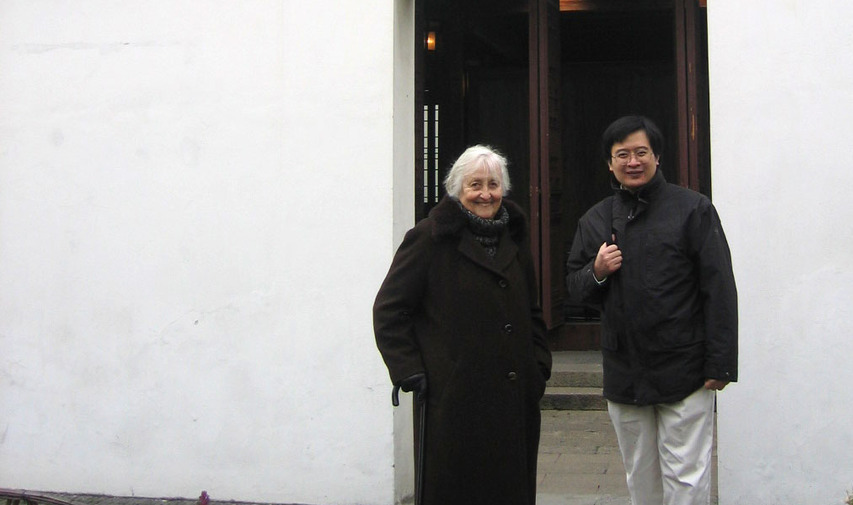# Professor Tong Yang 楊彤教授

Professor Tong Yang is a mathematician who proves the mathematical validity of equations underlying various physical laws that model real phenomena.

One of Professor Yang’s significant contributions is in the area of hyperbolic conservation laws, mathematical formulations of the principles of conservation of quantities such as mass and energy. Despite their widespread use in physics and engineering, the rigorous mathematical proof behind these laws remains incomplete. Solutions to hyperbolic equations include shock waves – mathematical discontinuities. These discontinuities pose mathematical difficulties; manipulations have to be performed to formulate weak solutions. The correctness of these solutions thus remains a contentious issue.

A key question in this research area concerns whether these equations are well-posed, meaning whether solutions for these equations exist, and whether such solutions are stable and unique. In 1999, Professor Yang offered an answer to this issue. Together with Professor Liu Taiping at Stanford University, he constructed the Liu-Yang functional, and established the well-posedness theory and stability of hyperbolic conservation laws in one-dimension.

Professor Yang has also researched on the Boltzmann equation in recent years. While conservation laws govern phenomena on a macroscopic scale, the Boltzmann equation describes the behaviors of gas molecules on the mesoscopic, or intermediate, scale. Professor Yang, in collaboration with four other mathematicians, proved that under a particular functional space, or a set of mathematical conditions, classical solutions to the Boltzmann equation without angular-cutoff exist. This established the well-posedness and regularity of the equation, thus solving a long-standing mathematical problem.

Professor Yang intends to use his fellowship to develop mathematical proofs for the Prandtl equations, fundamental equations that model phenomena under boundary layer conditions in fluid dynamics.

Professor Yang completed his PhD in Mathematics at the University of California, Davis in 1993, and received postdoctoral training at the Institute for Advanced Study, Princeton. He joined the faculty of the City University of Hong Kong in 1994, and was appointed to a professorship in 2002. He was awarded the Morningside Silver Medal of Mathematics in 1998. He is currently the Chair Professor of Mathematics at City University of Hong Kong.

### Current work

This year under the Croucher Senior Researcher Fellowship scheme, Professor Yang has been working on the following two problems: the hydrodynamic limit of the Boltzmann equation to compressible Euler equations, and the mathematical theories for Prandtl equation. Some progress has been made and details will be posted on the webpage later.